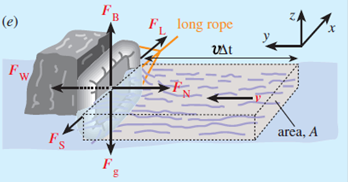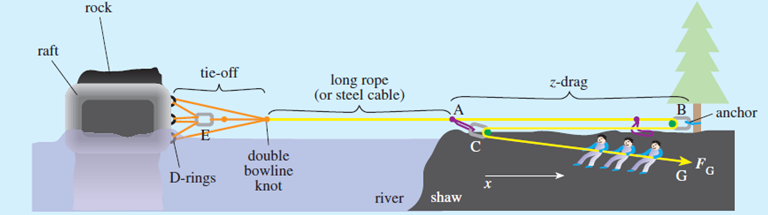Michael J. O’Shea   River Rescue Fluid flow, Newton’s second law and river rescue, M. J. O’Shea, Physics Education 41 137-143 (2006) A not-too-common occurrence (thankfully) involves a whitewater boat being pinned against a rock by the force of the moving water.  We estimate this force for various boats and look at a common mechanical advantage system (the Z-drag) used to extricate the boat from this situation.  Newton’s 2nd law is used to estimate the force of moving water on a wrapped kayak, canoe or raft.  A so-called ‘z-drag’ used in river rescue is analyzed and an example of a tie-off to a raft is also analyzed.  A raft is shown below being pushed sideways against a rock by moving water and several forces are present including the force of moving water FW and the force FL of a long rope used to attempt to extricate the boat.It can be shown via Newton’s 2nd law that FW ~ rAv2 where A is the perpendicular area of the boat exposed to the current and v is the speed of the current.  It’s important to note that this force depends on the speed of the current squared so if the current is doubled, then the force increases by a factor of four.  For the case where a raft with submerged area 4.4 m2  is being hit by a current of about 1.5 m/s, a force of 9.9 kN is obtained – a very substantial force!  To pull the boat off the rock a sideways pull would have to overcome the friction force between the boat material and the rock.  Assuming a static friction coefficient of 0.71 (rough estimate), the maximum value of static friction is 7.13 kN.  Thus one would have to pull sideways on the rope with a force greater than this amount to break the static friction and slide the raft off the rock.Since the Z-drag has a 3:1 mechanical advantage, the pull on the other end of the Z-drag would need to be about 2.4 kN.   This is still a pretty substantial force and would require 5 – 6 people.  Note: we have assumed ·        no friction in the rope system – this is a good approximation if high quality pulley wheels are used ·        all ropes in the z-drag are in-line A problem involving pulling a pinned kayak off a rock is here.  A series of photos of whitewater situations is shown here. A spreadsheet to allow calculation of forces and other interesting quantities for whitewater situations is here.